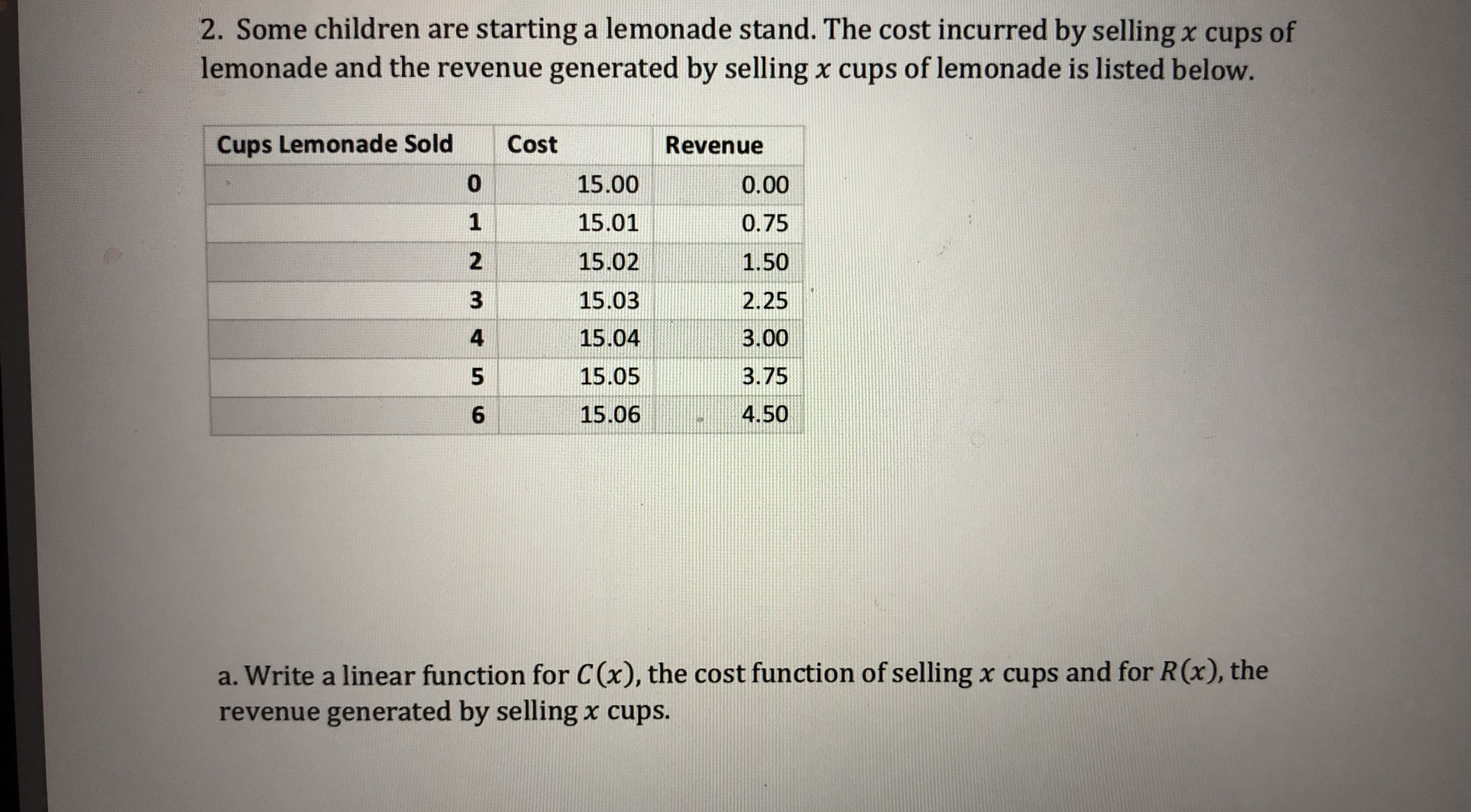# 2. Some children are starting a lemonade stand. The cost incurred by selling x cups oflemonade and the revenue generated by selling x cups of lemonade is listed below.Cups Lemonade SoldCostRevenue15.0015.0115.0215.0315.0415.0515.0600.000.751.502.253.003.754.50246a. Write a linear function for C(x), the cost function of selling x cups and for R(x), therevenue generated by selling x cups.

Question
30 viewshelp_outlineImage Transcriptionclose2. Some children are starting a lemonade stand. The cost incurred by selling x cups of lemonade and the revenue generated by selling x cups of lemonade is listed below. Cups Lemonade Sold Cost Revenue 15.00 15.01 15.02 15.03 15.04 15.05 15.06 0 0.00 0.75 1.50 2.25 3.00 3.75 4.50 2 4 6 a. Write a linear function for C(x), the cost function of selling x cups and for R(x), the revenue generated by selling x cups. fullscreen
check_circle

Step 1

Let us denote the cost of selling x cups that is C(x) by y.

Let the linear equation which describes the relationship between x and y is shown on board,where m is slope of line and b is y-intercept.

Step 2

The points that lie on the line y=mx+b are on the board.

Step 3

Slope of line can be calculated by taking the any two points on line&...

### Want to see the full answer?

See Solution

#### Want to see this answer and more?

Solutions are written by subject experts who are available 24/7. Questions are typically answered within 1 hour.*

See Solution
*Response times may vary by subject and question.
Tagged in

### Applications of Mathematics